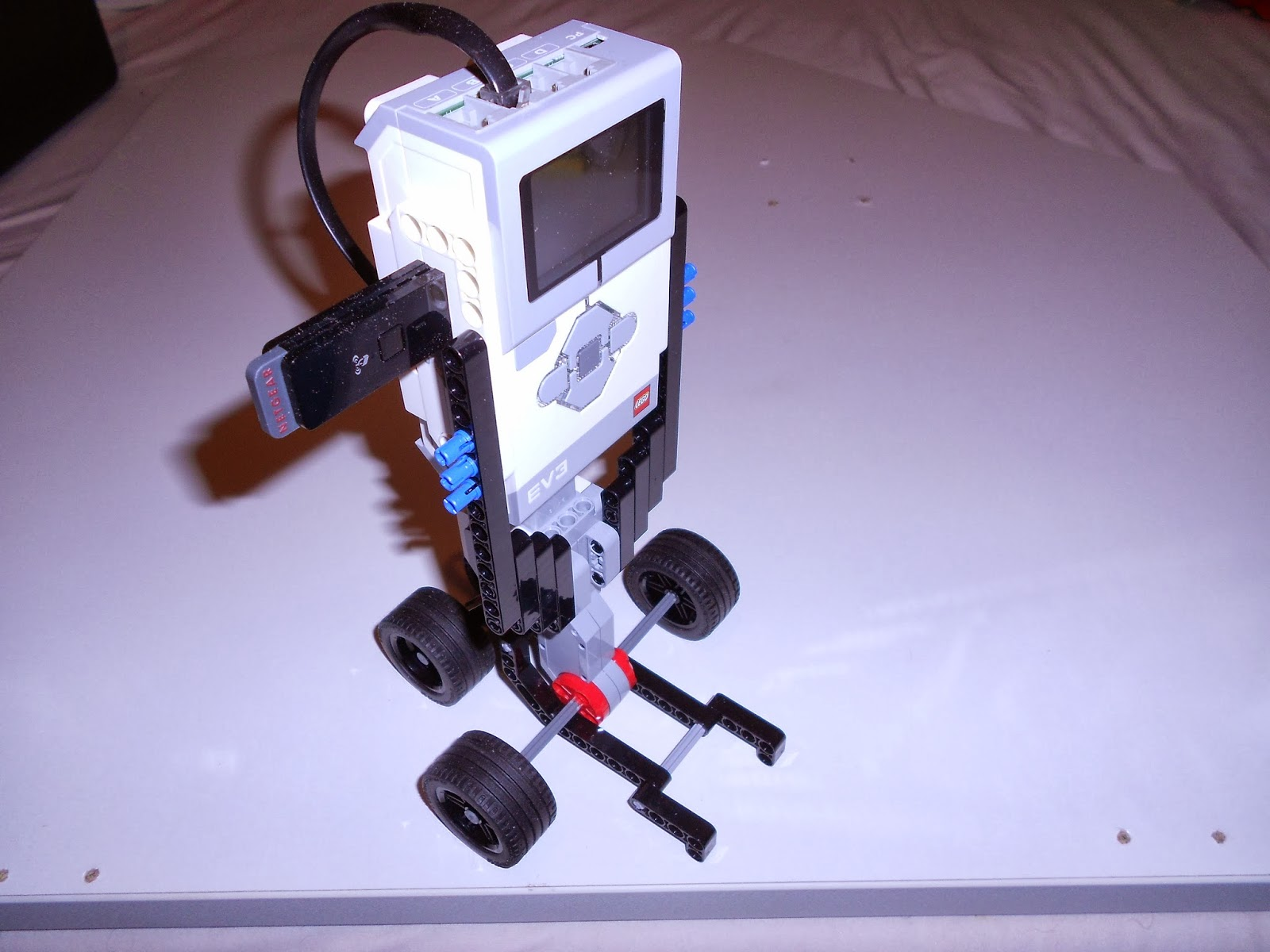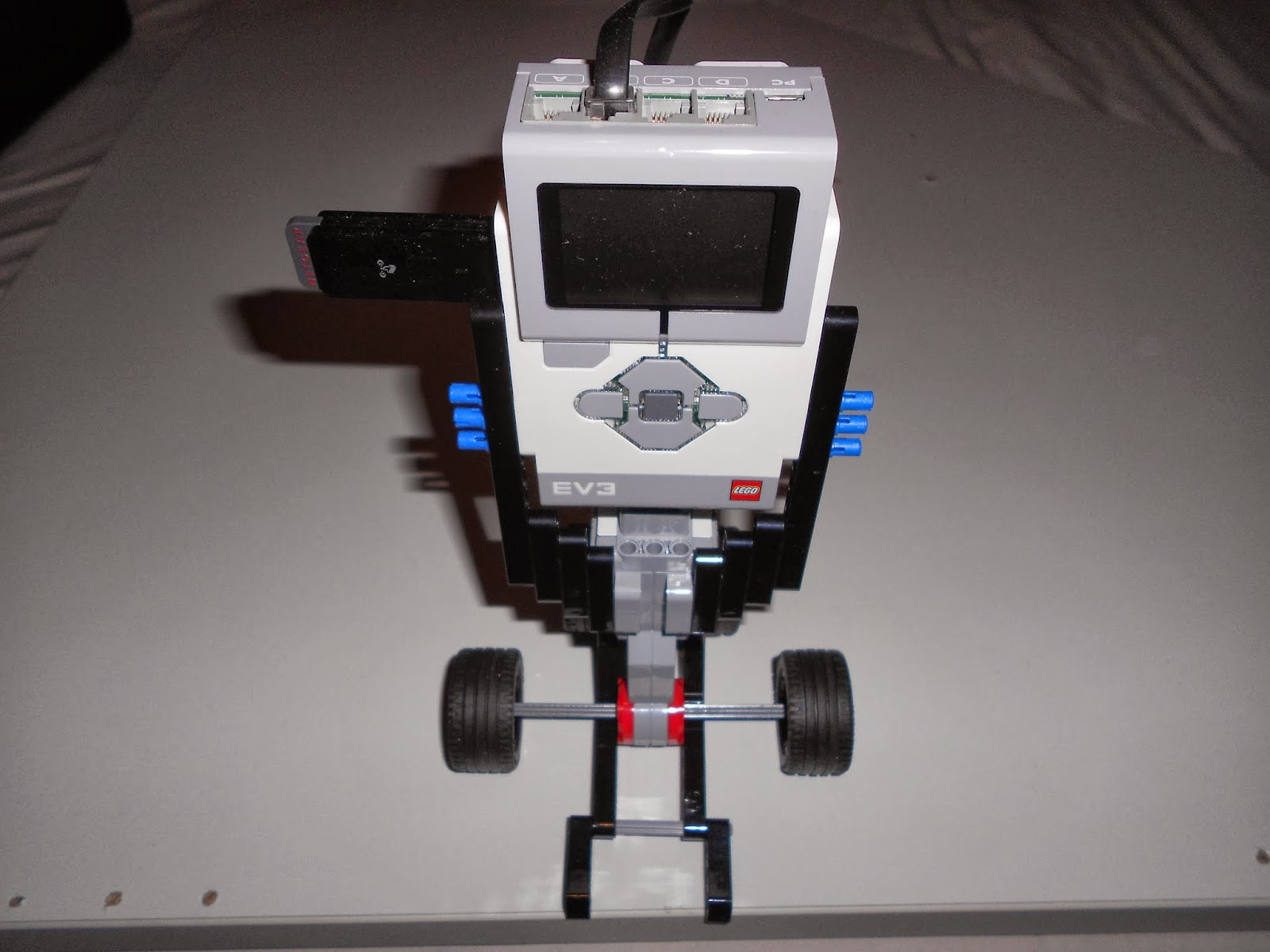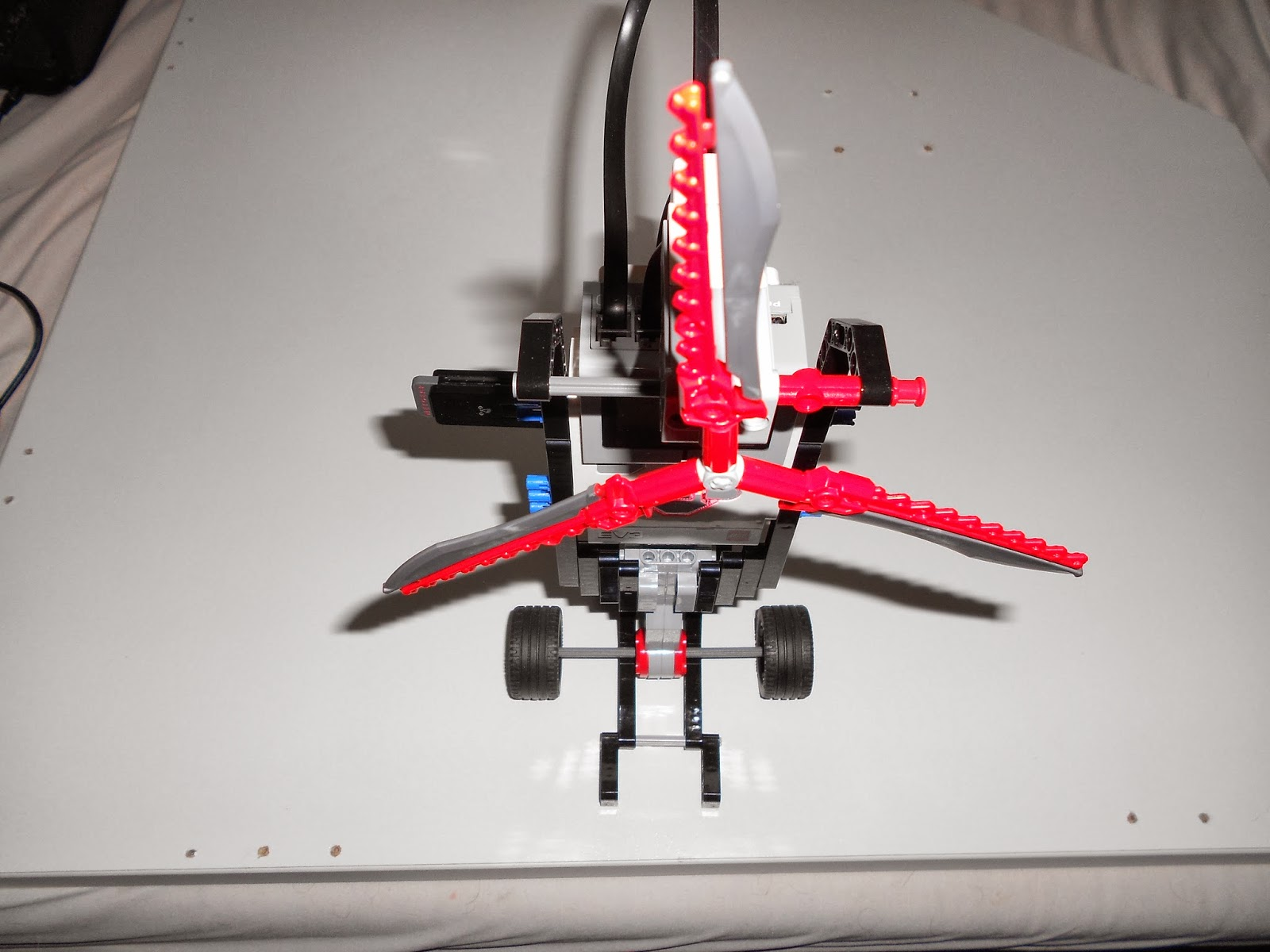### Lego Mindstorms EV3 Components: Motors - Part 1

With this posting I will start a series of articles where I explain which components/sensors are contained in the Lego Mindstorms EV3-set an how to work with them.

In this posting I will start with one of the most basic components - the motors. The Mindstorms-set includes three motors: two of the same size and a smaller one.

Both types work the same way and so they have the same api-methods.

I will build a simple program were I let the robot perform some movements to different directions.

Before we can start developing, we first need a robot which we will control with the program. I built a simple robot for that. It consists of only one single component connected to the brick: One of the bigger motors. There are two wheels plugged into the motor and two more wheels which aren't connected to the motor and just work as stabilization. Here are some pics:( There are different ways at different abstract levels to control the motors, but I will use the most basic method by using the Motor-class directly in this first part. We couldn't use a more abstract method which enable features like driving turns or following paths because we only have a single motor connected to our robot here. So the robot can just move forwards and backwards. )

I plugged the motor into plug connection B on the brick.

So, now we are ready to develop the program for our new robot. I set up an IntelliJ-project as described in our posting about developing leJOS programs with IntelliJ on Windows.

First, here is the plain code which is quite easy to understand:

```import lejos.hardware.motor.Motor;
import lejos.utility.Delay;

public class Main {
public static void main(String[] args) {

Motor.B.setAcceleration(200);
Motor.B.setSpeed(90);
Motor.B.rotate(360);
Motor.B.setSpeed(180);
Motor.B.rotate(360);
Motor.B.setSpeed(360);
Motor.B.rotate(360);
Motor.B.backward();
Delay.msDelay(3000);
Motor.B.stop();

}
}
```
What does that code do?
We address the motor in plug connection B via Motor.B. Then we set its acceleration to 200. The standard value is 6000 which means that the motor will accelerate by 6000 degrees per second per second. This is quite fast and so we lowered the value to 200 to get a smoother acceleration.
Then we set the speed of the motor to 90 degrees per second. After we "configured" the motor, we let it start rotating by 360 degrees. This operation is a blocking operation which means that the program will stop here until the motor rotated 360 degrees (which will be done after 4 seconds at the configured speed of 90 degrees per second).
After the motor rotated 360 degrees we double the speed to 180 degrees per second and let the motor rotate 360 degrees again. This time the motor will need half the time (2 seconds), of course.
After that the same happens again with a speed of 360 degrees per second.
Then, as the last part of the program, we call the backward-method. In contrast to the rotate-method, this method is non-blocking. That means, that the program will continue directly after calling that method, it won't wait till the backwards-movement is done (otherwise the motor wouldn't stop rotating anymore, there is no parameter for defining how long the motor should rotate). Recognize that there is also a rotate()-method with a second, boolean parameter. If you use that one with the boolean parameter = true the method becomes non-blocking like backward().
In the next line we tell the program to do nothing for 3 seconds, the motor will of course continue rotating (at the speed which was set as last = 360 degrees per second) during that time.
Then after these 3 seconds we let the motor stop und the program is at its end.

To get some feedback on what the robot is exactly doing through the program execution, we added some printlns to the console.

```import lejos.hardware.motor.Motor;
import lejos.utility.Delay;

public class Main {
public static void main(String[] args) {
/*
The standard value for acceleration is (6000 degrees per second) per second
which results in a very jerky start when the motor starts rotating.
To make the start smoother, the acceleration is set to a lower level.
*/
Motor.B.setAcceleration(200);

Motor.B.setSpeed(90);
System.out.println("Set speed to 90 degrees per second.");
System.out.print("Rotating 360 degrees...");
Motor.B.rotate(360);
System.out.println("done!");

Motor.B.setSpeed(180);
System.out.println("Set speed to 180 degrees per second.");
System.out.print("Rotating 360 degrees...");
Motor.B.rotate(360);
System.out.println("done!");

Motor.B.setSpeed(360);
System.out.println("Set speed to 360 degrees per second...");
System.out.print("Rotating 360 degrees...");
Motor.B.rotate(360);
System.out.println("done!");

Motor.B.backward();
System.out.print("Now rotating backwards for 3s");
Delay.msDelay(3000);
System.out.print("...3s are over...");
Motor.B.stop();
System.out.println("and stopped!");

}
}
```
That code looks better now, but there's tons of duplicate code. So we put the code for setting different speeds and letting the motor rotate in a function.

```import lejos.hardware.motor.Motor;
import lejos.utility.Delay;

public class Main {
public static void main(String[] args) {
/*
The standard value for acceleration is (6000 degrees per second) per second
which results in a very jerky start when the motor starts rotating.
To make the start smoother, the acceleration is set to a lower level.
*/
Motor.B.setAcceleration(200);

rotateDegreesAtSpeed(360, 90);
rotateDegreesAtSpeed(360, 180);
rotateDegreesAtSpeed(360, 360);

Motor.B.backward();
System.out.print("Now rotating backwards for 3s");
Delay.msDelay(3000);
System.out.print("...3s are over...");
Motor.B.stop();
System.out.println("and stopped!");

}

private static void rotateDegreesAtSpeed(final int degreesToRotate, final int degreesPerSecond) {
Motor.B.setSpeed(degreesPerSecond);
System.out.println("Set speed to "+degreesPerSecond+ "degrees per second.");
System.out.print("Rotating "+ degreesToRotate + "degrees...");
Motor.B.rotate(degreesToRotate);
System.out.println("done!");
}
}```

That was it. After transfering the program onto the brick (via WiFi) we executed the program. Here's a video of how the result looks like (console output readable in higher qualities).

Perfect. The next thing we did was extending the robot by a second motor - the smaller one. The result looked like the following:So the second motor will make the three "razorblades" rotate ( some eyecandy :) ).
We put the second motor in plug connection A of the brick.

The aim for that second motor should be: The razorblades should always rotate the same speed, the first motor has.

So we extended our program. Here's the finished code:
```import lejos.hardware.motor.Motor;
import lejos.utility.Delay;

public class Main {
public static void main(String[] args) {

final int ACCELERATION = 200;
final int ONE_ROTATION = 360;
/*
The standard value for acceleration is (6000 degrees per second) per second
which results in a very jerky start when the motor starts rotating.
To make the start smoother, the acceleration is set to a lower level.
*/
Motor.B.setAcceleration(ACCELERATION);
Motor.A.setAcceleration(ACCELERATION);

Motor.A.forward();
rotateDegreesAtSpeed(ONE_ROTATION, 90);
rotateDegreesAtSpeed(ONE_ROTATION, 180);
rotateDegreesAtSpeed(ONE_ROTATION, 360);

Motor.A.backward();
System.out.println("Switched Motor A from forward to backward. Waiting for the direction change.");
Delay.msDelay(2000);
Motor.B.backward();

System.out.print("Now rotating backwards for 3s");
Delay.msDelay(3000);
System.out.print("...3s are over...");
Motor.B.stop();
Motor.A.stop();
System.out.println("and stopped!");

}

private static void rotateDegreesAtSpeed(final int degreesToRotate, final int degreesPerSecond) {
Motor.A.setSpeed(degreesPerSecond);
Motor.B.setSpeed(degreesPerSecond);
System.out.println("Set speed of motors to "+degreesPerSecond+ " degrees per second.");
System.out.print("Rotating "+ degreesToRotate + " degrees...");
Motor.B.rotate(degreesToRotate);
System.out.println("done!");
}
}```
At the beginning of the program we set the acceleration of motor A to 200, too. Then we call Motor.A.forward(), which is a non-blocking operation like the backward()-method. We added one line in the created function to set the speed for motor A which is the same as for motor B, of course. After the three function calls we first call bachwards() on motor A. This will make the motor stop as the first step, and then start rotating backwards in a second step. Recognize, that Motor.A.backward() will be called when motor B stopped rotating. To let the robot wait until motor A also stopped, we added a delay of two seconds. After these two seconds the robot will start turning backwards (motor B will rotate into the other direction). At the same time, motor A will start rotating in the other direction, too.
We will let the robot move backwards for three seconds and then stop both motors.

So, here is the final result:

In the next article I will use the DifferentialPilot-class, which is the superior level of abstraction for controlling the motors, to move a two-wheeled robot which can drive turns for example.